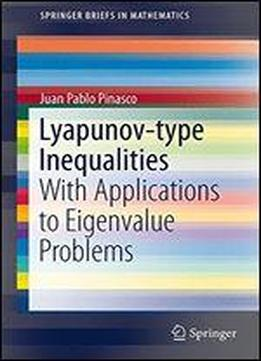# Lyapunov-type Inequalities: With Applications To Eigenvalue Problems by Juan Pablo Pinasco / 2013 / English / PDF

The eigenvalue problems for quasilinear and nonlinear operators present many differences with the linear case, and a Lyapunov inequality for quasilinear resonant systems showed the existence of eigenvalue asymptotics driven by the coupling of the equations instead of the order of the equations. For p=2, the coupling and the order of the equations are the same, so this cannot happen in linear problems. Another striking difference between linear and quasilinear second order differential operators is the existence of Lyapunov-type inequalities in R^n when p>n. Since the linear case corresponds to p=2, for the usual Laplacian there exists a Lyapunov inequality only for one-dimensional problems. For linear higher order problems, several Lyapunov-type inequalities were found by Egorov and Kondratiev and collected in On spectral theory of elliptic operators, Birkhauser Basel 1996. However, there exists an interesting interplay between the dimension of the underlying space, the order of the differential operator, the Sobolev space where the operator is defined, and the norm of the weight appearing in the inequality which is not fully developed. Also, the Lyapunov inequality for differential equations in Orlicz spaces can be used to develop an oscillation theory, bypassing the classical sturmian theory which is not known yet for those equations. For more general operators, like the p(x) laplacian, the possibility of existence of Lyapunov-type inequalities remains unexplored.

views: 205